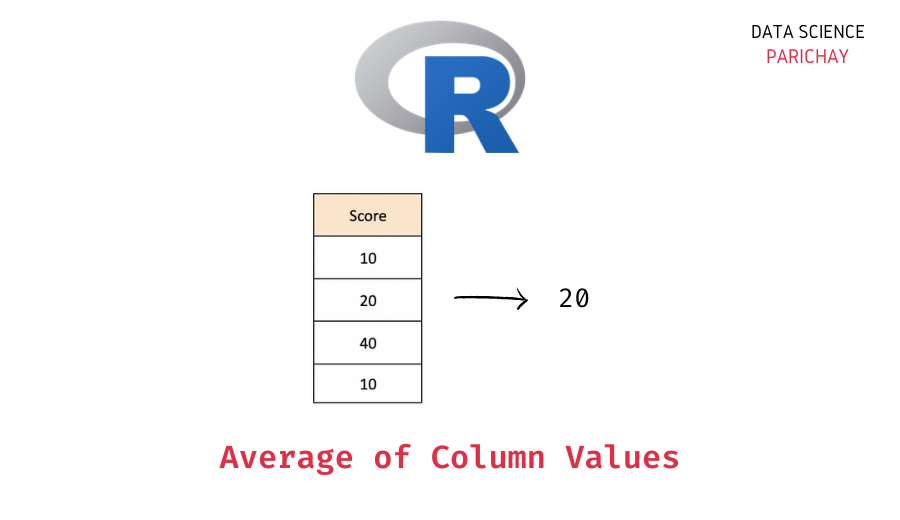# Average of Values in an R Column

The R programming language comes with a number of helpful functions to work with the data stored in data structures like vectors, lists, dataframes, etc. In this tutorial, we will look at one such function that helps us get the average of the values in a column of an R dataframe.

## How to get the average of a column in R?

You can use the built-in `mean()` function in R to compute the average of values in a dataframe column. Pass the column values as an argument to the function.

The following is the syntax –

`mean(dataframe[[column_name]])`

Pass `na.rm=TRUE` to avoid the `NA` values when computing the mean.

It returns the average of the values in the passed column.

## Steps to compute the average of values in an R column

Let’s now look at a step-by-step example of using the above syntax to compute the mean of a numeric column in R.

### Step 1 – Create a dataframe

First, we will create an R dataframe that we will be using throughout this tutorial.

```# create a dataframe
scores_df = data.frame(
"Team_A"= c(70, 80, 90),
"Team_B"= c(65, 95, 91)
)
# display the dataframe
print(scores_df)```

Output:

📚 Data Science Programs By Skill Level

Introductory

Intermediate ⭐⭐⭐

🔎 Find Data Science Programs 👨‍💻 111,889 already enrolled

Disclaimer: Data Science Parichay is reader supported. When you purchase a course through a link on this site, we may earn a small commission at no additional cost to you. Earned commissions help support this website and its team of writers.

```  Team_A Team_B
1     70     65
2     80     95
3     90     91```

We now have a dataframe containing the scores of two teams during a three-match basketball tournament. The dataframe has the columns – “Team_A” and “Team_B”. The values in each column represent the scores by the respective team.

### Step 2 – Calculate the average of values in the column using the `mean()` function

To calculate the average of values in a column, pass the column values as an argument to the `mean()` function. You can use the `[[]]` notation to access the values of a column.

Let’s compute the average points scored by both teams.

```# average of values in "Team_A"
avg_A = mean(scores_df[["Team_A"]])
# average of values in "Team_B"
avg_B = mean(scores_df[["Team_B"]])
# display the totals
print(avg_A)
print(avg_B)```

Output:

``` 80
 83.66667```

We get the average values in the “Team_A” column and the “Team_B” column.

## Average of a column with `NA` values in R

What if there are `NA` values in a column?

Let’s find out.

```# create a dataframe
scores_df = data.frame(
"Team_A"= c(70, NA, 90),
"Team_B"= c(65, 95, 91)
)
# display the dataframe
print(scores_df)```

Output:

```  Team_A Team_B
1     70     65
2     NA     95
3     90     91```

Here, we created a new dataframe such that one column contains `NA` and the other doesn’t contain any `NA` values.

Now, let’s apply the `mean()` function to both columns and compare the results.

```# average of values in "Team_A"
avg_A = mean(scores_df[["Team_A"]])
# average of values in "Team_B"
avg_B = mean(scores_df[["Team_B"]])
# display the totals
print(avg_A)
print(avg_B)```

Output:

``` NA
 83.66667```

We get `NA` as the average for the column with `NA` values and the actual average for the column without `NA` values. This happened because performing any mathematical operation with `NA` results in an `NA` in R.

If you want to compute the average of a column with `NA` values, pass `na.rm=TRUE` to the `mean()` function to skip the `NA` values when computing the mean.

```# average of values in "Team_A"
avg_A = mean(scores_df[["Team_A"]], na.rm=TRUE)
# average of values in "Team_B"
avg_B = mean(scores_df[["Team_B"]])
# display the totals
print(avg_A)
print(avg_B)```

Output:

``` 80
 83.66667```

We now get the average for the “Team_A” column excluding the `NA` values.

## Summary – Average of Column Values in R

In this tutorial, we looked at how to compute the average of a column in an R dataframe. The following is a short summary of the steps –

1. Create a dataframe (skip this step if you already have a dataframe on which you want to operate).
2. Use the `mean()` function to compute the average of the column values.
3. If your column contains any `NA` values, pass `na.rm=TRUE` to the `mean()` function to compute the average excluding the `NA` values in the column.

You might also be interested in –

•